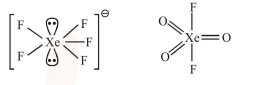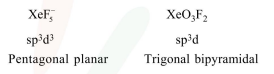# The shape/structure of [XeF5] and XeO3F2, respectivel. are:

Question:

The shape/structure of $\left[\mathrm{XeF}_{5}\right]^{-}$and $\mathrm{XeO}_{3} \mathrm{~F}_{2}$, respectively, are :

1. pentagonal planar and trigonal bipyramidal

2. trigonal bipyramidal and pentagonal planar

3. octahedral and square pyramidal

4. trigonal bipyramidal and trigonal bipyramidal

Correct Option: 1

Solution: